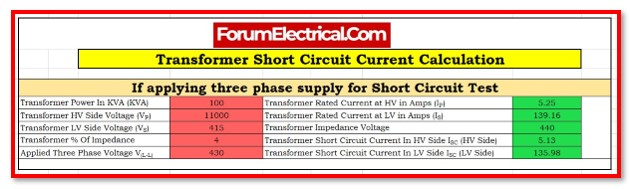# Transformer Short Circuit Current Calculation

0
286Electrical power distribution networks are subject to experiencing short circuits, which are often referred to as faults.

A fault current, also known as short circuit current, is the current that flows through a transformer whenever there is a defect on the load side of the transformer.

This fault currents, which are substantially larger than the rated current, are a condition that transformers have been constructed to be able to tolerate.

It is important to keep considering that the impedance of the transformer serves as one of the limiting factors for short circuit current that the transformer experiences at the secondary coil.

The ratio of fault current to rated current is decreased by a transformer that has a larger impedance, measured in percent Z. To explain it a different way, a Transformer that has a greater %Z restricts the flow of fault current more effectively.

## Formula

The formula described below is used to determine the short circuit current of the transformer in HV and LV side.

### Step 1

Obtain the following information

• KVA rating of the transformer
• Secondary Voltage
• % Impedance (from the transformer’s name plate, assume 5%)

### Step 2

IP= KVA X 1000/ 1.732 X VP

IS= IP X (VP/VS)

VIMPEDENCE = (% of Impedance/100) x Vp

### Step 3

Determine the short circuit current.

Transformer Short Circuit Current in HV Side

ISC (HV Side)  = IP /ISx V(L-L)

Transformer Short Circuit Current in LV Side

ISC (LV Side)= (VP/VS) x ISC (HV Side) .

Therefore, this is the current generated by the short circuit. Users may use this information to determine the rating of the circuit breaker.